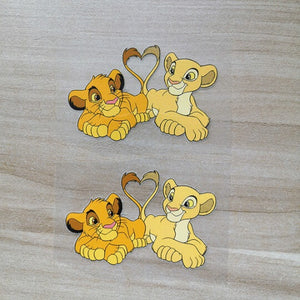" .... The Lion King Simba and Nala Iron On Stickers for Decal Your Kid Shoes – theshoesgirl
Order Over \$120 can cover free FedEx shipping!
Order Over \$120 can cover free FedEx shipping!# The Lion King Simba and Nala Iron On Stickers for Decal For Your Kid Shoes

Regular price \$15.00 \$9.90 Save \$5.10

ONLY 519583 LEFT

 Hours   Minutes   Seconds

Sale Ends Once The Timer Hits Zero!

ONLY 519583 LEFT

Get these easy use iron on the Lion King Simba and Nala stickers to make a unique gift for your kid shoes. Best size for DIY or custom  Air Force 1 & Vans.

<<<<<<<<<<<<<<<<<<<<<<<<<<<<<<<<<<<<<<<<<<<<<<<<<<<<<<<

Why use these the Lion King patches:

• 1. Perfect sizes for sneakers and other items, such as socks, hoodies, jeans, jackets....
• 2. Waterproof and flexible, when iron on, proved durable after washing test and brushing test. You can check the videos at the bottom of front page or my YouTube Channel: www.youtube.com/c/theshoesgirl
• 3. Easy use, just iron on directly, no need any transfer paper, no need any sealer. Will not peel off even brushing in water! You can watch the test in my videos.
• 4. Durable but still can be removed, if you are tired with this design after several months, remove them and change another design to refresh your shoes.

<<<<<<<<<<<<<<<<<<<<<<<<<<<<<<<<<<<<<<<<<<<<<<<<<<<<<<<

• ### The set includes:

• 2 sheets of Simba and Nala
<<<<<<<<<<<<<<<<<<<<<<<<<<<<<<<<<<<<<<<<<<<<<<<<<<<<<<<
• ### Size:

width: 7.5cm; height: 4.5 cm

<<<<<<<<<<<<<<<<<<<<<<<<<<<<<<<<<<<<<<<<<<<<<<<<<<<<<<<

### How to use:

1. Position the the Lion King patches on the shoes with tape, mark on the tape, then cut along with the marks.

2. Iron on the rest part of the patches, please keep temperature 135-150℃

3. peel the vinyl, the image of the Lion King patches will stick on the shoes.

4. If use normal iron, please turn off the steam, keep the temperature above, use the tip and be careful and patient, for it's heavy to control, better use the mini iron.

5. Watch the tutorial video here: